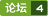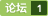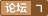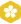### 完成端口接受数据丢失 [问题点数：40分，结帖人myfriend023]WSARecv rnrnWSABUF的len 是用来控制接收多少字节吗？rnrn那假设这里 len 是50 ，但实际套接字上只有20字节<em>数据</em>，那么<em>完成</em><em>端口</em>会收到<em>完成</em>通知包么？

rnrn我有<em>完成</em><em>端口</em>的问题，我的基本模型是一个聊天室rnrn当收到一个消息的时候就全盘发出去（所有的客户端socket我都保存在一个list中）rn当接收到一个send结束的消息后，投送一个recv，基本上可以正常工作，问题在于当某个socket推出的时候，他会收到很多的推出信息（也就是<em>接受</em>的字节数=0地<em>完成</em>通告），很烦，想了很多办法都不行，不知道该如何解决，

1. 创建并绑定到设备IO   BOOL Create(int nMaxConcurrency = 0) { m_hIOCP = CreateIoCompletionPort( INVALID_HANDLE_VALUE, NULL, 0, nMaxConcurrency); chASSERT(m_hIOCP != NULL); return(

<em>完成</em><em>端口</em>封装成一个类来使用，简单容易扩展．

rn这个是Repository的 用的JPArn[code=java]@Query(value =" WITH cte1 AS (select to_char(created_time,'YYYY-MM') AS months from e_expenseformitem WHERE created_time BETWEEN '2019-01-01' and '2019-07-31' group by months ),\n" +rn "cte2 AS (\n" +rn "SELECT\n" +rn "\tto_char ( created_time, 'YYYY-MM' ) AS months,\n" +rn "\tsum( fill_figure ) AS train_amount \n" +rn "FROM\n" +rn "\te_expenseformitem i \n" +rn "WHERE\n" +rn "\tsubject_name LIKE '%火车%' \n" +rn "GROUP BY\n" +rn "\tmonths \n" +rn "\t),\n" +rn "\tcte3 AS (\n" +rn "SELECT\n" +rn "\tto_char ( created_time, 'YYYY-MM' ) AS months,\n" +rn "\tsum( fill_figure ) AS plane_amount \n" +rn "FROM\n" +rn "\te_expenseformitem i \n" +rn "WHERE\n" +rn "\tsubject_name LIKE '%飞机%' \n" +rn "GROUP BY\n" +rn "\tmonths \n" +rn "\t),\n" +rn "\tcte4 AS (\n" +rn "SELECT\n" +rn "\tto_char ( created_time, 'YYYY-MM' ) AS months,\n" +rn "\tsum( fill_figure ) AS car_amount \n" +rn "FROM\n" +rn "\te_expenseformitem i \n" +rn "WHERE\n" +rn "\tsubject_name LIKE '%自驾%' \n" +rn "GROUP BY\n" +rn "\tmonths \n" +rn "\n" +rn "\t),\n" +rn "\tcte5 AS (\n" +rn "SELECT\n" +rn "\tto_char ( created_time, 'YYYY-MM' ) AS months,\n" +rn "\tsum( fill_figure ) AS hotel_amount \n" +rn "FROM\n" +rn "\te_expenseformitem i \n" +rn "WHERE\n" +rn "\tsubject_name LIKE '%住宿%' \n" +rn "GROUP BY\n" +rn "\tmonths \n" +rn "\n" +rn "\t),\n" +rn "\tcte6 AS (\n" +rn "SELECT\n" +rn "\tto_char ( created_time, 'YYYY-MM' ) AS months,\n" +rn "\tsum( fill_figure ) AS cl_amount \n" +rn "FROM\n" +rn "\te_expenseformitem i \n" +rn "WHERE\n" +rn "\tsubject_name LIKE '%材料%' \n" +rn "GROUP BY\n" +rn "\tmonths \n" +rn "\n" +rn "\t),\n" +rn "\tcte7 AS (\n" +rn "SELECT\n" +rn "\tto_char ( created_time, 'YYYY-MM' ) AS months,\n" +rn "\tsum( fill_figure ) AS kd_amount \n" +rn "FROM\n" +rn "\te_expenseformitem i \n" +rn "WHERE\n" +rn "\tsubject_name LIKE '%快递%' \n" +rn "GROUP BY\n" +rn "\tmonths \n" +rn "\n" +rn "\t),\n" +rn "\tcte8 AS (\n" +rn "SELECT\n" +rn "\tto_char ( created_time, 'YYYY-MM' ) AS months,\n" +rn "\tsum( fill_figure ) AS zd_amount \n" +rn "FROM\n" +rn "\te_expenseformitem i \n" +rn "WHERE\n" +rn "\tsubject_name LIKE '%招待费%' \n" +rn "GROUP BY\n" +rn "\tmonths \n" +rn "\n" +rn "\t),\n" +rn "\tcte9 AS (\n" +rn "SELECT\n" +rn "\tto_char ( created_time, 'YYYY-MM' ) AS months,\n" +rn "\tsum( fill_figure ) AS fz_amount \n" +rn "FROM\n" +rn "\te_expenseformitem i \n" +rn "WHERE\n" +rn "\tsubject_name LIKE '%房租%' \n" +rn "GROUP BY\n" +rn "\tmonths \n" +rn " \n" +rn "\t),\n" +rn "\tcte10 AS (\n" +rn "SELECT\n" +rn "\tto_char ( created_time, 'YYYY-MM' ) AS months,\n" +rn "\tsum( fill_figure ) AS tx_amount \n" +rn "FROM\n" +rn "\te_expenseformitem i \n" +rn "WHERE\n" +rn "\tsubject_name LIKE '%通讯%' \n" +rn "GROUP BY\n" +rn "\tmonths \n" +rn "\n" +rn "\t),\n" +rn "\tcte11 AS (\n" +rn "SELECT\n" +rn "\tto_char ( created_time, 'YYYY-MM' ) AS months,\n" +rn "\tsum( fill_figure ) AS water_amount \n" +rn "FROM\n" +rn "\te_expenseformitem i \n" +rn "WHERE\n" +rn "\tsubject_name LIKE '%水费%' \n" +rn "GROUP BY\n" +rn "\tmonths \n" +rn " \n" +rn "\t),\n" +rn "\tcte12 AS (\n" +rn "SELECT\n" +rn "\tto_char ( created_time, 'YYYY-MM' ) AS months,\n" +rn "\tsum( fill_figure ) AS df_amount \n" +rn "FROM\n" +rn "\te_expenseformitem i \n" +rn "WHERE\n" +rn "\tsubject_name LIKE '%电费%' \n" +rn "GROUP BY\n" +rn "\tmonths \n" +rn "\n" +rn "\t),\n" +rn "\tcte13 AS (\n" +rn "SELECT\n" +rn "\tto_char ( created_time, 'YYYY-MM' ) AS months,\n" +rn "\tsum( fill_figure ) AS cw_amount \n" +rn "FROM\n" +rn "\te_expenseformitem i \n" +rn "WHERE\n" +rn "\tsubject_name LIKE '%财务%' \n" +rn "GROUP BY\n" +rn "\tmonths \n" +rn "\n" +rn "\t),\n" +rn "\tcte14 AS (\n" +rn "SELECT\n" +rn "\tto_char ( created_time, 'YYYY-MM' ) AS months,\n" +rn "\tsum( fill_figure ) AS bg_amount \n" +rn "FROM\n" +rn "\te_expenseformitem i \n" +rn "WHERE\n" +rn "\tsubject_name LIKE '%办公%' \n" +rn "GROUP BY\n" +rn "\tmonths \n" +rn "\n" +rn "\t) SELECT\n" +rn "\ta.months,\n" +rn "\tb.train_amount,\n" +rn "\tc.plane_amount,\n" +rn "\td.car_amount,\n" +rn "\te.hotel_amount,\n" +rn "\tf.cl_amount,\n" +rn "\tg.kd_amount,\n" +rn "\th.zd_amount,\n" +rn "\ti.fz_amount,\n" +rn "\tj.tx_amount,\n" +rn "\tk.water_amount,\n" +rn "\tl.df_amount,\n" +rn "\tm.cw_amount,\n" +rn "\tn.bg_amount \n" +rn "FROM\n" +rn "\tcte1 a\n" +rn "\tLEFT JOIN cte2 b ON a.months = b.months\n" +rn "\tLEFT JOIN cte3 c ON a.months = c.months\n" +rn "\tLEFT JOIN cte4 d ON a.months = d.months\n" +rn "\tLEFT JOIN cte5 e ON a.months = e.months\n" +rn "\tLEFT JOIN cte6 f ON a.months = f.months\n" +rn "\tLEFT JOIN cte7 g ON a.months = g.months\n" +rn "\tLEFT JOIN cte8 h ON a.months = h.months\n" +rn "\tLEFT JOIN cte9 i ON a.months = i.months\n" +rn "\tLEFT JOIN cte10 j ON a.months = j.months\n" +rn "\tLEFT JOIN cte11 k ON a.months = k.months\n" +rn "\tLEFT JOIN cte12 l ON a.months = l.months\n" +rn "\tLEFT JOIN cte13 m ON a.months = m.months\n" +rn "\tLEFT JOIN cte14 n ON a.months = n.months\n" +rn "\t\n" +rn "ORDER BY months",nativeQuery = true)rn List findTatalChart();rnrn这个是serviceImplrnrn@Overridern public List findTotalChart() rn List tatalChart = expenseFormItemRepository.findTatalChart();rn for (Object[] objects : tatalChart) rn System.out.println(">>>>>>>>>>>>>>>>>>>>>>>>>>>>>");rn Object o = JSON.toJSON(objects);rn System.out.println(o);rn rn return tatalChart;rn [/code]rnrnrn打印出来的结果为rn["2019-01",null,null,30.0,4340.0,null,790.0,1921.0,null,149.0,null,44.64,null,449.17]rnrn["2019-02",null,null,null,2510.0,null,1513.0,782.0,1000.0,647.5,null,71.75,null,4106.8099999999995]rnrn["2019-03",null,null,null,8370.0,null,1559.0,4260.0,6883.0,149.7,null,206.64,null,2341.28]rnrn["2019-04",null,null,5031.0,1080.0,null,118.0,855.0,null,null,null,null,null,204.76]rnrn["2019-05",null,null,null,null,null,null,null,null,null,null,null,null,null]rnrn["2019-06",null,null,286.8,180.0,null,37.0,null,1111.0,null,null,null,null,null]rnrn["2019-07",null,null,12.0,null,null,10.0,null,500.0,null,null,null,null,null]rnrn但是<em>数据</em>库查出来的是[img=https://img-bbs.csdn.net/upload/201907/05/1562294276_295772.png][/img]rn后台打印的<em>数据</em>少了很多 ，比如五月份的都是null ，求助~

<em>完成</em><em>端口</em>，下载文件的代码。用vs2015实现，想了解<em>完成</em><em>端口</em>的可以学习了结一下。下载步骤包括：登录-》版本验证-》有新版本就下载，没有新版本就不下载，已经下载了也不下载，登录失败也不下载。
flash 数据丢失

printf数据丢失。。。。。。。。。。。。。。。
int main()rnrn char a,b;rnrn strcpy(a,"00");rn strcpy(b,"00"); rn printf("%s------%s\n",a,b); rn rn return 0；rnrnrn输出结果：------00rnrn请问，a的值哪去了？

rt
RabbitMQ数据丢失
1）生产者弄丢了<em>数据</em> 生产者将<em>数据</em>发送到rabbitmq的时候，可能<em>数据</em>就在半路给搞丢了，因为网络啥的问题，都有可能。 解决： RabbitMQ提供transaction和confirm模式来确保生产者不丢消息。transaction机制就是说，发送消息前 开启事物(channel.txSelect())，然后发送消息，如果发送过程中出现什么异常， 事物就会回滚(channel.txR...

<em>数据</em>库在一台机器上 然后好多人在用 下午 我在做项目的时候 发现原来的<em>数据</em>库里面的一个值变了 原来是‘ex1’ 变成3+. 然后我就把值改过来看看 但是发现 出现了“在进行流水过程 事务无法执行” 但是我运行了下 还是改好了 但是问题是过了不久 发现这个<em>数据</em>库的 一些表的值都没有了 只剩下空表 请问是不是我的问题呀 这个问题是为什么呢！
vfp数据丢失
use data\holidayrnuse data\newholidayrnappend from data\holiday for appbydate=='同意'.and. days<>'U'rnuse rnusernclose allrnuse data\holidayrndele allrnpackrnclose allrnmessagebox("报往人事部请假成功",64,"eton group")rnrnrn这些代码用行时，有时候szholiday一条<em>数据</em>都没有,有时候都在(我的前提是holiday是有<em>数据</em>的)rnrn请问是什么问题?rn谢谢rn
redis数据丢失

2-pandas-数据丢失

class Vertex;rnrnclass Edgernrn Vertex* vertexes;rn Edge(Vertex* one, Vertex* two);rnrnclass Facernrn Vertex* vertexes;rn Edge* edges;rn Face(Vertex*one, Vertex* two, Vertex* three);rn setEdge(Edge *e， int count);//edge[count] = e;rnrnmain()rnrn vertexList.push_back(vertexOne);rn vertexList.push_back(vertexTwo);rn vertexList.push_back(vertexThree); vertexIter = vertexList.begin();rn rn Edge e1(vertexList, vertexList + 1);rn Edge e2(vertexList + 1, vertexList + 2);rn Edge e3(vertexList, vertexList + 2);rn rn Face face(vertexIter, vertexIter + 1, vertexIter + 2);rn edgeIter = edgeList.begin();rn edgeList.push_back(e1);rn face.setEdge(edgeIter);rn edgeList.push_back(e2);rn face.setEdge(edgeIter + 1); //此时 会出现edges消失的现象rn edgeList.push_back(e3);rn face.setEdge(edgeIter + 2); //此时 仅有edges中的<em>数据</em>还存在rnrn创建3个vector分别存vertex、edge、face对象：vertexList、edgeList、faceListrn创建3个vertex对象存入vertexListrn然后利用vertexList的iterator创建3个edge对象，将他们存入edgeListrn最后创建Face对象face，通过setEdge，利用edgeList的iterator做指针，将edgeList中的三个边先后注入face中，rn这时出现了问题：注入第一个edges成功，但当我继续注入时，会出现face中的edges<em>丢失</em>的现象，rn最后仅仅edges中还有值，请问这是怎么回事，是由于何处生成了临时变量吗，求解释

Sub combc()rn pdhz = 1rn TIM_F2 = Nowrn If Hour(TIM_F2) = 7 Thenrn Ado_hzb.RecordSource = "select * from hzc_hz"rn Ado_hzb.Refreshrn Ado_pdhz.RecordSource = "select jldbh,ghdwbm,shdwbm,wzbm,c_erp01,c_erp02,sum(ljll) as ljhz from hzc_table " & _rn " where year(jjrq)='" & Year(Now) & "' and month(jjrq)='" & Month(Now) & _rn "' and day(jjrq)='" & Day(Now) & "' and (datepart(hh,jjrq)>=0 and datepart(hh,jjrq))的<em>数据</em>和那个sql语句查出来的<em>数据</em>不一样，总是丢数，是什么原因呢？

utf-8 数据丢失

if(write(socket_id,send_data,strlen(send_data),0)==-1)rnrn //error();rnrnelsernrn //把send_data写入文件rn｝rn但是我发现文件里send_data,是完整的，长度为１０．rn为什么ethareal,抓包工具，抓到的长度只有４，？rn期待高手讲解，谢谢rn

kafka数据丢失

asp.net 数据丢失

printf数据丢失-------------
int main()nn char a,b;nn strcpy(a,"00");n strcpy(b,"00"); n printf("%s------%s\n",a,b); n n return 0；nnn输出结果：------00nn求解，a的值哪去了？

access数据丢失

Flash数据丢失
STM32在干扰环境下的Flash<em>数据</em><em>丢失</em>，官方发布的一个文档

baud=57600,每秒传输6k<em>数据</em>时出现<em>数据</em><em>丢失</em>现象，rn机器配置为c1G, 我采用事件触发异步模式采集，如果提高通讯线程的优先级，rn<em>数据</em>一般不会丢，而用普通优先级的线程，经常会出现<em>数据</em><em>丢失</em>的现象rn不知什么原因，我觉得普通级的也应该够用了。
mscomm数据丢失
MSCOMM，在9600波特率下，用二进制方式接收，<em>数据</em>老是<em>丢失</em>。请教如何解决

%Iterative learning control for mobile robot path-trackingrnclear all;rnclose all;rnrnts=0.001; %Sample timernfor k=1:1:2001rn xd(k)=cos((k-1)*pi*ts);rn yd(k)=sin((k-1)*pi*ts);rn thd(k)=ts*pi*(k-1)+pi/2;rnendrnrnrnfor k=1:1:2001rn u1(k)=0;u2(k)=0;rn e1(k)=0;e2(k)=0;rn e3(k)=0;rnendrny0=[1;0;pi/2];rn%%%%%%%%%%%%%%%%%%%%%%%%%%%%%%%%%%%%%%%%%%%%%%%%%%%%%%%%%%%rnM=300;rnfor i=0:1:M % Start Learning Control for M Timesrnirnpause(0.05);rnrn%%%%%%%%%%%%%%%%%%%%%%%%%%%%rnfor k=1:1:2001rnif k==1rn q=y0;rnendrnxp(k)=q(1);rnyp(k)=q(2);rnth(k)=q(3);rnqd=[xd(k);yd(k);thd(k)];rnce1(k)=qd(1)-q(1); % Current error of time krnce2(k)=qd(2)-q(2); rnce3(k)=qd(3)-q(3); rnu=[u1(k);u2(k)];rnB=ts*[cos(q(3)) 0rn sin(q(3)) 0 rn 0 1];rnL1=0.10*[cos(q(3)) sin(q(3)) 0;rn 0 0 1];rnL2=L1;rnb1=0.02;b2=0.03;%<em>数据</em><em>丢失</em>rnrnrnK1=L1;K2=L2*b1*b2-L1; rnrncond=norm((eye(3)+B*K2)^(-1)*(eye(3)-B*K1)); % Conditions: cond must be smaller than 1.0rnrnU=u+K1*[ce1(k+1);ce2(k+1);ce3(k+1)]+K2*[ce1(k);ce2(k);ce3(k)];rnu1(k)=U(1);rnu2(k)=U(2);rnu=[u1(k);u2(k)];rnq=q+B*u;rnrnrnend % End of krnfigure(1);rnhold on;rnplot(xd,yd,'r',xp,yp,'b');rnxlabel('xd xp');ylabel('yd,yp');rnrnj=i+1;rntimes(j)=j-1;rne1i(j)=max(abs(ce1));rne2i(j)=max(abs(ce2));rne3i(j)=max(abs(ce3));rnend %End of irnfigure(2);rnplot(xd,yd,'r',xp,yp,'b');rnxlabel('xd xp');ylabel('yd,yp');rnfigure(3);rnplot(times,e1i,'*-r',times,e2i,'o-b',times,e3i,'o-k');rntitle('Change of maximum absolute value of e1,e2 and angle with times i');rnxlabel('times');ylabel('e1,e2 and angle'); rn显示 Attempted to access ce1(2); index out of bounds because numel(ce1)=1.rnrnError in ==> Untitled5 at 51rnU=u+K1*[ce1(k+1);ce2(k+1);ce3(k+1)]+K2*[ce1(k);ce2(k);ce3(k)]rn这种要怎么改

if(write(socket_id,send_data,strlen(send_data),0)==-1)rnrn //error();rnrnelsernrn //把send_data写入文件rn｝rn但是我发现文件里send_data,是完整的，长度为１０．rn为什么ethareal,抓包工具，抓到的长度只有４，？rn期待高手讲解，谢谢rn

Exchange 2013 是微软新的企业级邮件解决方案，同时也是一个安全和高效地协作平台，本课程将从零开始，详尽说明 Exchange 2013 的相关功能和配置，为您展示 Exchange 2013 的强大功能和优秀设计，使您能掌握 Exchange 2013 的部署和配置。

<em>完成</em><em>端口</em>服务器端的封装. 主要提供了一个TIOCPServer类. 其用法参见单元说明.
c++ 完成端口

IOCP 完成端口
<em>完成</em><em>端口</em>实现源码~~~~~~~~~~~~~~~~~~~~~~~~~~~~~~~~~~~~~~~~~~~~~~~~~~~~~

<em>完成</em><em>端口</em>实际上是一个通知队列，当某项I/O操作<em>完成</em>时，由操作系统向<em>完成</em><em>端口</em>发送通知包。该通知包包含的信息有传输的字节数、<em>完成</em>键和重叠结构。点击跳转至示例代码实现<em>完成</em><em>端口</em>模型的基本步骤：1.创建<em>完成</em><em>端口</em>调用CreateIoCompletionPort函数来创建<em>完成</em><em>端口</em>对象，其原型如下：HANDLE WINAPI CreateIoCompletionPort( _In_ HANDLE Fi

VC<em>完成</em><em>端口</em>商调用GetQueuedCompletionStatus返回FALSE，GetLastError返回64（网络名不可用），使用移动GPRS网络经常出现这个问题，但是使用TCP网线连接基本上不会有这个问题。rnrn这个到底是怎么回事情！64错误，我一定要关闭socket吗？如果有其它原因造成64错误，有什么办法避免。解决问题就结帖。